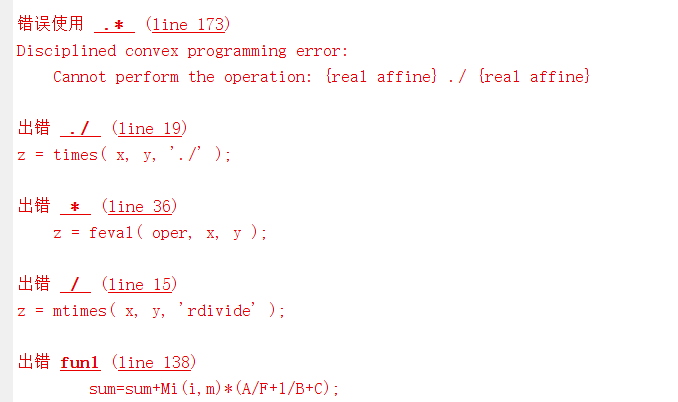Here’s my variable and constraint, and it’s telling me I’m making an error
variable a0(i);
variable am(i,m);
variable w0(i,m);
variable wc(i,m);
variable v(i,m);

A=am(i,m);
D=C(i,m)/Req(i,3);
E=am(i,m)*lmd(i);
F=D-E;
B=v(i,m);
C=wc(i,m)Req(i,4);
sum=sum+Mi(i,m)
(A/F+1/B+C);%This is line 138Have you read the CVX user’s Guide? If so, you would not have seen any allowance for dividing affine expressions.

Have you proven your optimization problem is convex? You haven’t shown the entirety of your problem, and you seem to not be showing us an intended for loop, so forum readers don’t know what use is made of 'sum`, so we can’t be sure there is not a convex reformulation, but the burden is on you to show convexity.